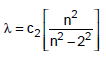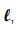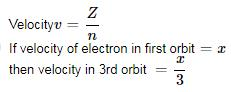Courses

# Atomic Structure (Chapter Test - Non-Medical)

## 20 Questions MCQ Test Chemistry for IIT JEE (Main & Advanced) | Atomic Structure (Chapter Test - Non-Medical)

Description
This mock test of Atomic Structure (Chapter Test - Non-Medical) for Class 12 helps you for every Class 12 entrance exam. This contains 20 Multiple Choice Questions for Class 12 Atomic Structure (Chapter Test - Non-Medical) (mcq) to study with solutions a complete question bank. The solved questions answers in this Atomic Structure (Chapter Test - Non-Medical) quiz give you a good mix of easy questions and tough questions. Class 12 students definitely take this Atomic Structure (Chapter Test - Non-Medical) exercise for a better result in the exam. You can find other Atomic Structure (Chapter Test - Non-Medical) extra questions, long questions & short questions for Class 12 on EduRev as well by searching above.
QUESTION: 1

Solution:
QUESTION: 2

Solution:
QUESTION: 3

### The radii of two of the first four Bohr’s orbits of the hydrogen atom are in the ratio 1 : 4. The energy difference between them may be

Solution:
QUESTION: 4

The number of radial nodes of 3s and 2p-orbitals are respetively

Solution:
QUESTION: 5

α-Particles are ..... times heavier than neutron

Solution:
QUESTION: 6

An excited hydrogen atom emits a photon of wavelength λ in returning to the ground state. If R is the Rydberg constant than the quantum number n of the excited state is

Solution:
QUESTION: 7

A proton and an α-particle are accelerated through the same potential difference. The ratio of their de Broglie wavelength is

Solution:
QUESTION: 8

In an atom two electrons move around the nucleus in circular orbits of radii R and 4R. The ratio of the time taken by them to complete one revolution is

Solution:
QUESTION: 9

The circumference of nth orbit in H-atom can be expressed in terms of de Broglie wavelength λ as

Solution:
QUESTION: 10

The two particles A and B have de Broglie wavelengths 1 nm and 5 nm respectively. If mass of A is four times the mass of B, the ratio of kinetic energies would be

Solution:
QUESTION: 11

How fast is an electron moving, if, it has a wavelength equal to the distance it travels in 1 sec ?

Solution:
QUESTION: 12

One requires energy En to remove a nucleon and an energy Ee to remove an electron from the orbit of an atom, then

Solution:
QUESTION: 13

Iffor Balmer series, what is the value of c2 ?

Solution:
QUESTION: 14

The magnetic moment order is correctly given in

Solution:
QUESTION: 15

The electron identified by quantum numbers n andI. n = 4, l = 1      II. n = 4, l = 0
III. n = 3, l = 2    IV. n = 3, l = 1

can be placed in order of increasing energy from the lowest to highest

Solution:
QUESTION: 16

The energy of an electron in the Bohr’s first orbit of Hatom is –13.6 eV. The possible energy value(s) of the excited state(s) for electrons in Bohr’s orbits of hydrogen is(are)

Solution:
QUESTION: 17

The orbital represented by ψ4,2,0 is

Solution:
QUESTION: 18

The angular momentum of electron in hydrogen atom is proportional to

Solution:
QUESTION: 19

If the speed of an electron in the Bohr’s first orbit of hydrogen atom bex , speed of the electron in 3rd orbit is

Solution:QUESTION: 20

When photons of energy 4.25 eV strike the surface of a metal A, the ejected photoelectrons have maximum kinetic energy, TA (expressed in eV) and de Broglie wavelength λA, The maximum kinetic energy of photoelectrons liberated from another metal B by photons of energy 4.70 V is TB = TA –1.50eV. If the de Broglie wavelength of these photoelectrons is λB = 2λA, then which is not correct?

Solution: